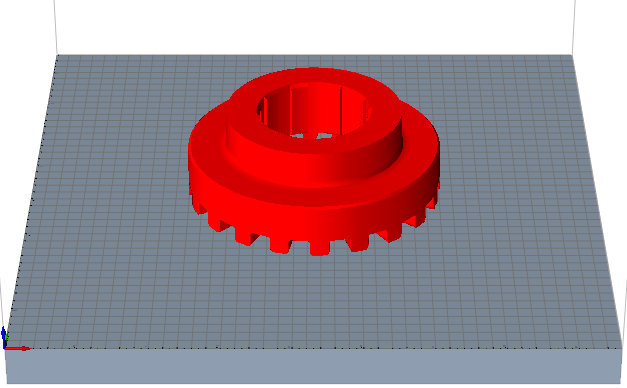# Result Types for Binder Sinter

3D printing analysis for Binder Sintering produces results for Displacement, Sinter Deviation, Relative Density, Temperature, and Volume Change.

## Displacement

The displacement result type shows how much the part’s shape will deform from its initial shape during the baking process. The areas of the part shown in the darkest orange will have the most displacement from the part’s initial shape. If the part was scaled (that is, one or more of the scale factors was set to >1), the displacement will be based on the scaled shape. If the initial part shape is the same as the desired final part shape, then this result will be identical to the Sinter Deviation result.

When reviewing displacement results, you should check that the order of magnitude of the displacement and the shape of the deformation make sense. Use the Animation tools on the Analysis Explorer to view the shape of the deformation. Setters can help mitigate the part’s displacement.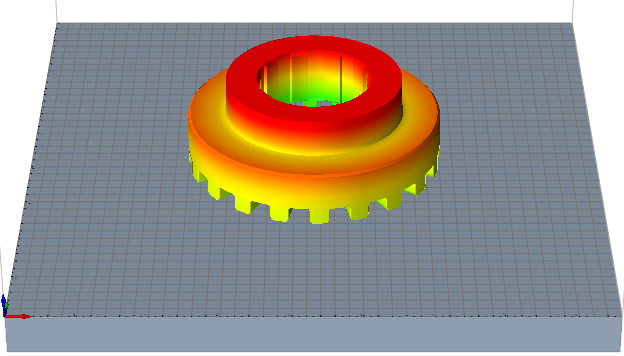## Sinter Deviation

This result shows the deviation of the part shape from the desired final part shape/dimensions.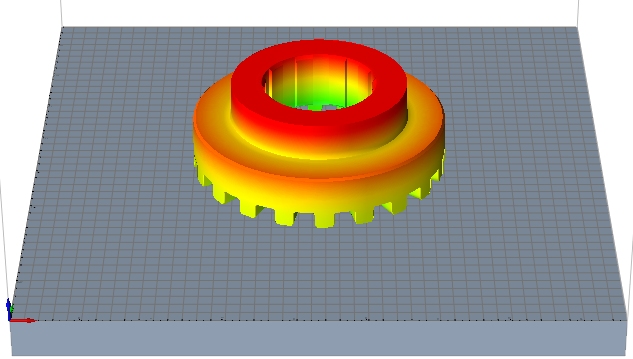## Relative Density

This result shows how the relative density of the part increases through the sintering process to reach maximum densification. The density of the part is presented through the ratio of the solid density and called relative density.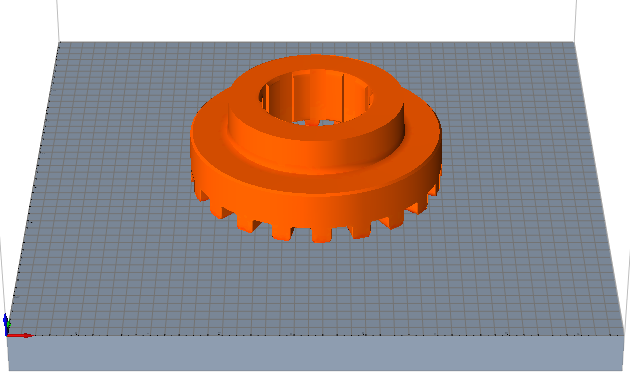## Temperature

This result type shows the part’s temperature at each node of the part during the sintering process.

The temperature of the part can be either computed using the law of conservation of energy or assigned as per the temperature curve. When the latter option is used, we assume the part is the same (uniform) temperature as the value in the curve.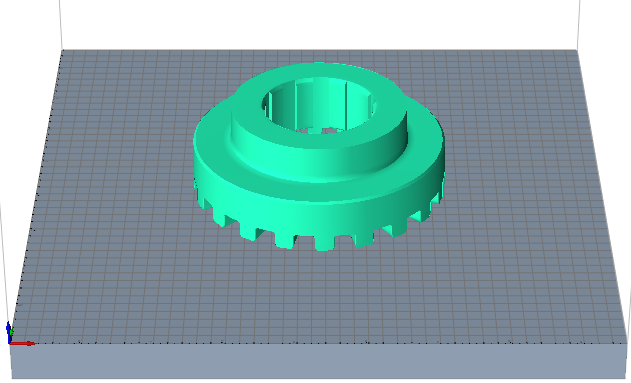## Volume Change

This result shows how the volume of each element changes during the sintering process. This will help to understand how the volume is changing locally.